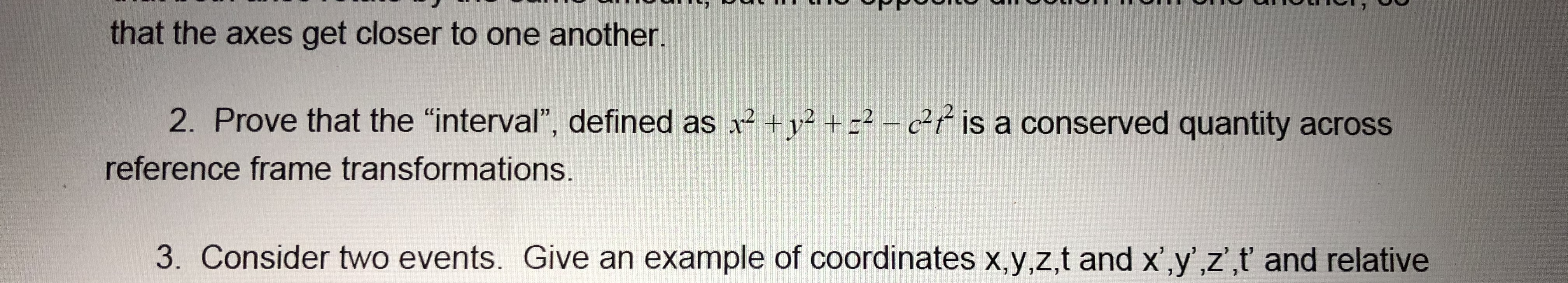# that the axes get closer to one another.2. Prove that the "interval", defined as x² +v² + =² – c²¢ is a conserved quantity acrossreference frame transformations.3. Consider two events. Give an example of coordinates x,y,z,t and x',y',z',t and relative

Question
2 viewshelp_outlineImage Transcriptionclosethat the axes get closer to one another. 2. Prove that the "interval", defined as x² +v² + =² – c²¢ is a conserved quantity across reference frame transformations. 3. Consider two events. Give an example of coordinates x,y,z,t and x',y',z',t and relative fullscreen
check_circle

star
star
star
star
star
1 Rating
Step 1

For the given problem, the invariance of the space-time interval has been considered for the transformations that take place between the inertial reference frames.

Let there be a stationary inertial reference frame defined by S, whose space coordinates are defined as
(x, y, z) and the time coordinate is defined as t.

Let there be another inertial reference frame defined by S’, whose space coordinates are defined as (x’, y’, z’) and the time coordinate is defined as t’.

Let the inertial frame S’ be moving at a constant velocity v along the x direction with respect to the stationary reference frame S.

Let the interval in the S frame be denoted as Δs.

This “interval” is mathematically expressed as,

Step 2

Consider an event that takes place in the S frame defined by the space time coordinates (x, y, z, t).

The corresponding event will transform in the moving S’ frame as per the Lorentz transformations.

Step 3

Let the interval in the S’ frame be denoted as Δs’

The correspondin...

### Want to see the full answer?

See Solution

#### Want to see this answer and more?

Solutions are written by subject experts who are available 24/7. Questions are typically answered within 1 hour.*

See Solution
*Response times may vary by subject and question.
Tagged in

### Science